# MCQ Questions for Class 6 Maths Chapter 13 Symmetry with Answers

Check the below NCERT MCQ Questions for Class 6 Maths Chapter 13 Symmetry with Answers Pdf free download. MCQ Questions for Class 6 Maths with Answers were prepared based on the latest exam pattern. We have provided Symmetry Class 6 Maths MCQs Questions with Answers to help students understand the concept very well. https://mcqquestions.guru/mcq-questions-for-class-6-maths-chapter-13/

Students can also refer to NCERT Solutions for Class 6 Maths Chapter 13 Symmetry for better exam preparation and score more marks.

## Symmetry Class 6 MCQs Questions with Answers

MCQ On Symmetry For Class 6 Question 1.
How many lines of symmetry does the figure have?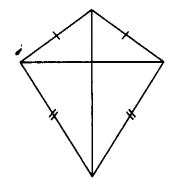(a) 1
(b) 2
(c) 3
(d) 4

Symmetry Class 6 MCQ Question 2.
How many lines of symmetry does the figure have?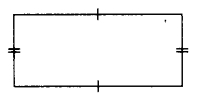(a) 1
(b) 2
(c) 3
(d) 4.

Symmetry MCQ Class 6 Question 3.
How many lines of symmetry does the figure have?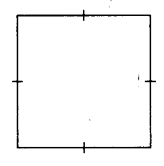(a) 1
(b) 2
(c) 3
(d) 4

MCQ Questions On Symmetry For Class 6 Question 4.
How many lines of symmetry does the figure have?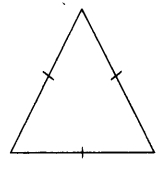(a) 1
(b) 2
(c) 3
(d) 4

MCQ Questions For Class 6 Maths Chapter 13 Question 5.
How many lines of symmetry does the figure have ?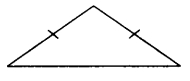(a) 1
(b) 2
(c) 3
(d) 4

Class 6 Maths Chapter 13 MCQ Question 6.
How many lines of symmetry does the figure have ?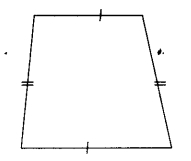(a) 1
(b) 2
(c) 3
(d) no line of symmetry

MCQ On Symmetry Class 6 Question 7.
How many lines of symmetry does the figure have?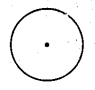(a) 1
(b) 2
(c) 3
(d) Countless.

Class 6 Symmetry MCQ Question 8.
How many lines of symmetry does the figure have?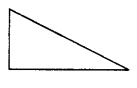(a) 0
(b) 1
(c) 2
(d) countless

MCQs On Symmetry For Grade 6 Question 9.
How many lines of symmetry does the figure have?(a) 1
(b) 2
(c) 3
(d) 4

Question 10.
How many lines of symmetry does a regular hexagon have?
(a) 1
(b) 3
(c) 4
(d) 6

Question 11.
Which of the following letters has horizontal line of symmetry?
(a) C
(b) A
(c) J
(d) L.

Question 12.
Which of the following letters has horizontal line of symmetry?
(a) Z
(b) V
(c) U
(d) E.

Question 13.
Which of the following letters has horizontal line of symmetry?
(a) S
(b) W
(c) D
(d) Y.

Question 14.
Which of the following letters has vertical line of symmetry?
(a) R
(b) C
(c) B
(d) T.

Question 15.
Which of the following letters has vertical line of symmetry?
(a) N
(b) K
(c) B
(d) M.

Question 16.
Which of the following letters has vertical line of symmetry?
(a) J
(b) D
(c) E
(d) O.

Question 17.
Which of the following letters has no line of symmetry?
(a) P
(b) O
(c) H
(d) X.

Question 18.
Which of the following letters has no line of symmetry?
(a) O
(b) X
(c) I
(d) Q